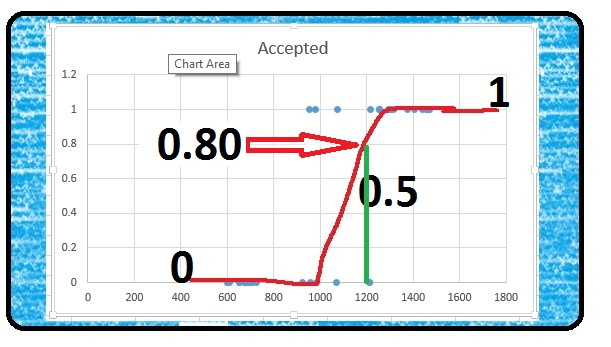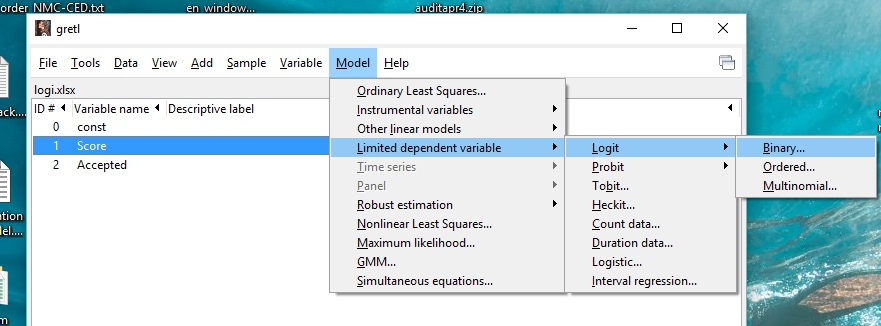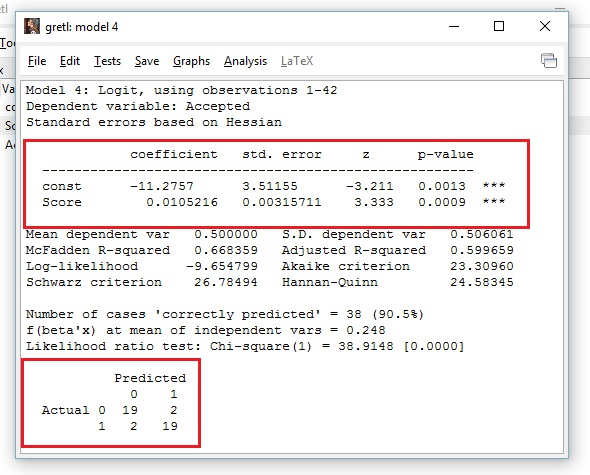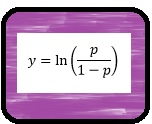# Logistic Regression with Gretl

One of the most popular machine learning algorithms, Logistic Regression is actually a classification algorithm. Broken down to its simplest terms, binary logistic regression (the one we will be focusing on here) is answering a yes or no question. Will the customer buy or not? Is the email SPAM or not?

## Score Accepted 982          0 1304         1 1256         1 1562         1 703          0

Above is a small sample from the data set we will be using for this lesson. In this set, student scores for an entrance test are listed in the first column and whether they were Accepted (1) or Not(0) is in the second column.

I ran a scatter plot on the data with Scores on the X axis. As you can see the dots for 2 horizontal lines at 1 and 0. You may notice that the 1 (Accepted) dots seem to cluster towards higher scores and 0 (Not Accepted) dots cluster towards lower scores.Well since the point of Logistic Regression is help us make predictions, here is how the predictions work. The Logistic Regression, represented by my crudely drawn red S, goes from 1 to 0. And just like with Linear Regression, if we take a value for X, to make our prediction, we look for the value of Y on the line at that point.In the case of a 1200 score, if we check the value of Y on the line, we get .80. This roughly translates to mean, that with a score of 1200, a student has an 80% chance of being accepted.

## Let’s meet Gretl

While there are third party add-ons you can download for Excel that will do Logistical Regression, in its native form, Excel does not do a good job in this area. So I thought this would be a great opportunity to introduce you to a neat piece of FREE software called Gretl.

So why Gretl? Why not R or Python? I mean those are the languages real data scientists use right?

That is true, and R and Python can easily do a Logical Regression. The problem is  however, in order to use R and Python, you need to know how to program. Gretl, on the other hand, is GUI based. Think of it as a point and click light weight R. It is no where near as robust as R, but for learning how to do Logistical Regression, Gretl does a fine job.

After you install and start Gretl, the next step is to load in the data. Go to File>Open Data>User File. Search for the Excel file you downloaded previously in this lesson. Make sure you then select Excel from the file type at the bottom of the screen.Select logi.xlsx. Leave the Start Import at window at 1 and 1. This is where the data starts in our Excel file: 1rst column, 1rst row. You will get a message letting you know how much data was imported.

The next pop up will noted that the data is undated. Click No on this window.You data columns (Score, Passed) will appear in the  Gretl window. If you click on one, the data from that column will appear in a pop-up window. **note in the file you download, column 2 will be Accepted not Passed.

## Let’s Model

Without further ado, let us do some modelings. From the menu bar Model>Limited dependent variable>Logit>Binary…Now you have to select you Dependent variable and Regressors. Here is a hint, the dependent variable is what we want to find. What are we looking for? Will the person be Accepted. So Accepted goes in Depentdent variable and Score goes in Regressors. Pick the Show p-values radio button and then click Okay.Below are the results of your Logistic Regression model

I am not going to give a Stats lesson here, but I will cover the important points.1. The top red box contains some important information. First the coefficients represent the b and m values from the linear equation we will be using later: y=mX+b =y=0.0105216X + -11.2757
2. The p-value of Score = 0.0009 This is important as the p-value is a probabilistic value  that determines whether or not the regressor variable truly affects the dependent variable. The most common p-value threshold you are likely to come across is 0.05. If your regressor variable has a p-value above 0.05, you will want to reconsider your model.
3. The matrix at the bottom of the screen. This shows you how successfully your model predicted outcomes from the training data set. It translates of the 0’s (not accepted) the model got 19 out of 21 right. For 1’s(accepted) the model got 19 out of 21 right. That is a 90% success rate. Not bad.

## Let’s Use the Model

Okay, so maybe you jumped ahead and tried 1200 in the linear formula we developed above. It is 1.325?? How is that? Isn’t this supposed to be between 0 and 1.

Well the problem is, we are not looking for Y we are looking for probability (p). Y in this case is not the Y intercept, but instead:Well, we know Y = 1.325 for a score of 1200, how do we find p from that? We solve  for p. Now feel free to go and do the math yourself if you want, but I already did the work for you. The equation below solves for p. If you don’t trust me and want to do it yourself, be my guest, but I assure you the equation below is good.## Let’s Make a Prediction

Let us put the formula’s we have found into ExcelNow you have a working prediction model. Any value you place in the score cell will be calculated to Y and p (probability). As the example above shows, a score of 1200 give us a probability of .79.

Turns out my crummy drawing wasn’t so bad after all.